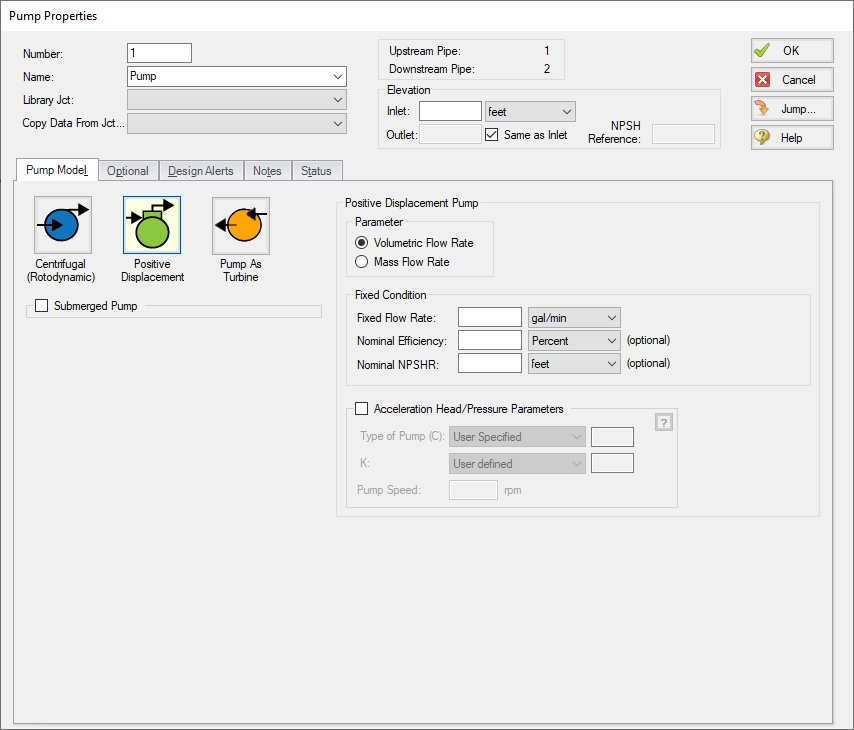### Positive Displacement Pump

Positive Displacement pumps can be used to provide a fixed flow for steady state analysis.

Parameter

• Volumetric Flow Rate - Fix the volumetric flow rate through the pump.

• Mass Flow Rate - Fix the mass flow rate through the pump.

Fixed Condition

• Fixed Flow Rate - The value and unit of the fixed flow amount.

• Nominal Efficiency - A fixed efficiency, useful to calculate power requirements. This is an optional input.

• Nominal NPSHR - Net Positive Suction Head Required for the pump at the specified flow. This is an optional input.

Acceleration head is the energy required to change the velocity of the fluid in the system from zero to some non-zero velocity. This requires additional energy beyond the amount required simply to overcome the frictional losses in a system. This is reflected when starting a centrifugal pump - the peak power requirement is higher than during steady operation. Similarly, positive displacement pumps are constantly accelerating and decelerating the fluid in the suction line. The value for acceleration head can be added to the Pump Summary via Output Control, and is visible in the Applied Standards tab.

• Type of Pump (C) - The type of Positive Displacement Pump, for the purposes of calculated the acceleration head pump type coefficient, as described below. If no predefined types are applicable, a user specified value must be entered.

• K - The compressibility factor of the fluid, as described below.

• Pump Speed - The nominal RPM of the Positive Displacement Pump.

This suction line acceleration head can by calculated with the method defined in ANSI/HI 6.1-6.5-2015ANSI/HI Standard 6.1-6.5-2015, American National Standard for Reciprocating Power Pumps for Nomenclature, Definitions, Application, and Operation, 2015, published by American National Standards Institute, 6 Campus Drive, Parsippany, NJ, USA. . This method requires the user definition of pump speed, pump type coefficient, and a fluid compressibility factor. Suction line length and fluid velocity are also required and are calculated by AFT Fathom.• hacc - Acceleration head (ft)

• l - Length of suction line (ft)

• v - Fluid velocity in suction line (ft/s)

• n - Pump speed (rpm)

• C - Coefficient determined by type of reciprocal pump:

• Simplex (Single-acting) = 0.628

• Simplex (Double-acting) = 0.200

• Duplex (Single-acting) = 0.200

• Duplex (Double-acting) = 0.115

• Triplex = 0.066

• Quintuplex = 0.040

• Septuplex = 0.028

• Nonuplex = 0.022

• K - Factor representing relative compressibility of fluid. Some common compressibility factors are provided.

• Hot Water = 1.4

• Hot Oil = 2.5

• g - Gravitational constant (ft/sec2)

AFT Fathom calculates the total acceleration head by summing the calculated acceleration head for each individual pipe. The solver will attempt to automatically determine the pipes in the suction line by tracing the flow upstream of the pump back to an Assigned Pressure or Reservoir junction. If at any point upstream of the pump there is more than one potential path (for example, two inflows merging together), this calculation will not proceed because the suction line is indeterminant. However, the flow can split into multiple outflows. As long as there is one continuous and unique inflow path to the pump, acceleration head can by calculated.

Note: Though the ANSI/HI 6.1-6.5-2015 standard states that the NPSHA should be corrected to account for acceleration head, Fathom will not adjust the NPSHA shown in the output. The user should subtract the acceleration head from the displayed NPSHA to comply with the standard.

Submerged Pump

Represents a pump with a fixed head or pressure at suction. See Submerged Pump.Figure 1: Positive Displacement Pump Model

Related Blogs